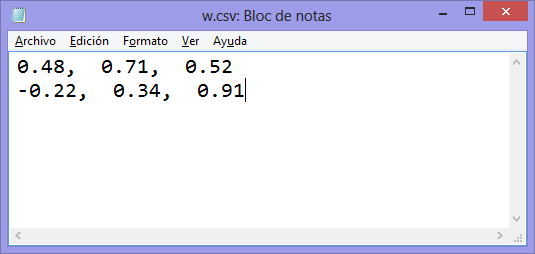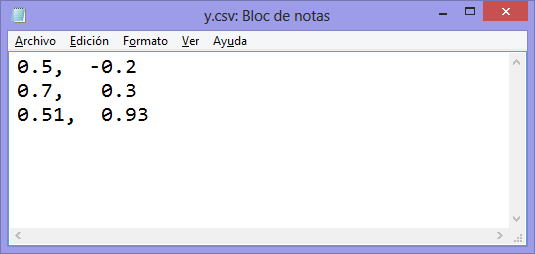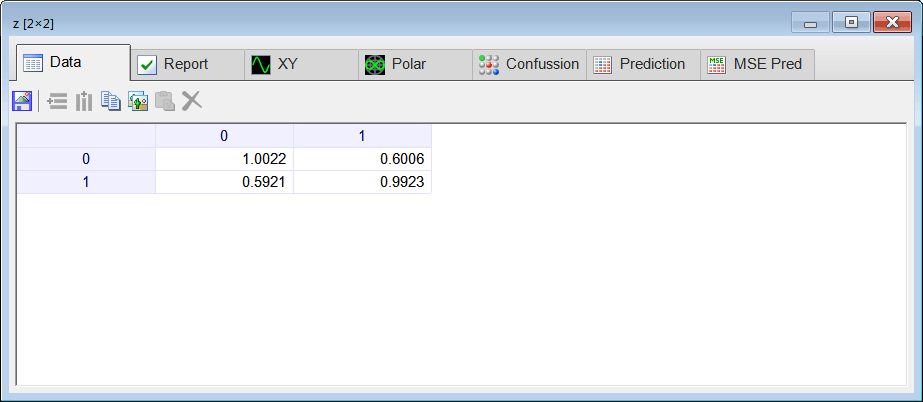# Introduction

## Supervised Training (Supervised Learning)

A multilayer ANN is trained using the input training set and the target training set. This implies that for each input there is an associated desired output typically called target. Thus, a multilayer ANN learns using supervised training.

## Unsupervised Training (Unsupervised Learning)

An artificial neural network can be used to discover hidden information in the data without relying on a target training set. This kind of networks are trained using unsupervised training because, the network decides by itself where the information is, and what information is important to perform the learning process.

 Tip To train an ANN using unsupervised training, it is necessary to have only the input training set. As there are not right results, no validation is required. This implies that once the network training error is acceptable, the training is acceptable. Thus, ANNs under unsupervised training may provide new insights about a subject where knowledge is limited.

## Kohonen Network

One ANN that uses unsupervised training is the Kohonen network invented by Teuvo Kohonen. This network is a two-layer network. Some types of networks have an extra input called synthetic input. The figure below shows a Kohonen network with two inputs and two outputs. Observe how the Input Normalization block produces an extra input y3. Observe also that the neurons do not have any activations function.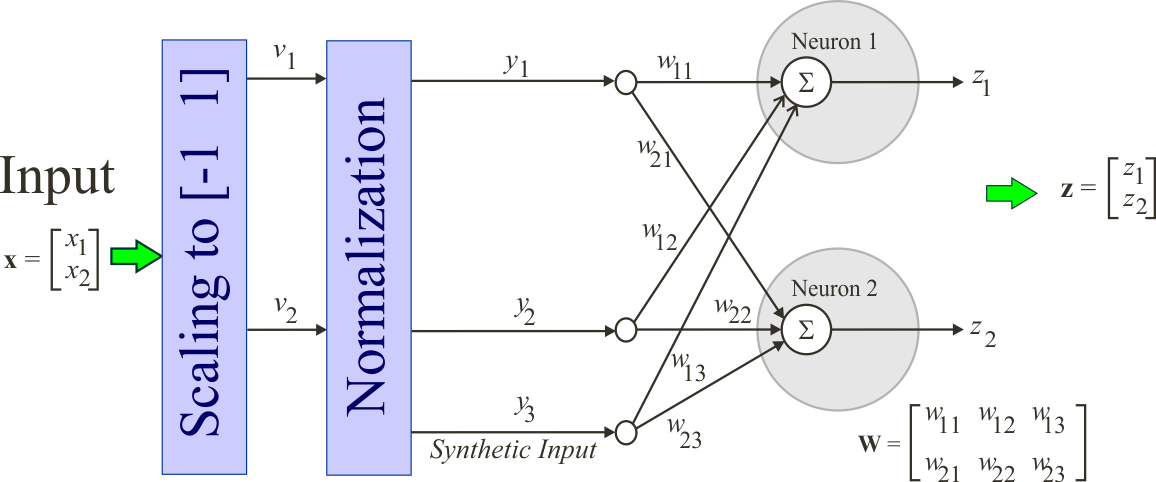## Input Scaling

A Kohonen network requires all input values to be in the range from -1 to 1. Typically, a linear transformation is applied to the raw data x to produce v. There are, however, other transformations that may be used as long as the values of v are in the range from -1 to 1.

 Problem 1 Compute manually the output of an Kohonen network when the normalized input y is: (a) y = [0.5, 0.7, 0.51 ], (b) y = [-0.2, 0.3, 0.93 ],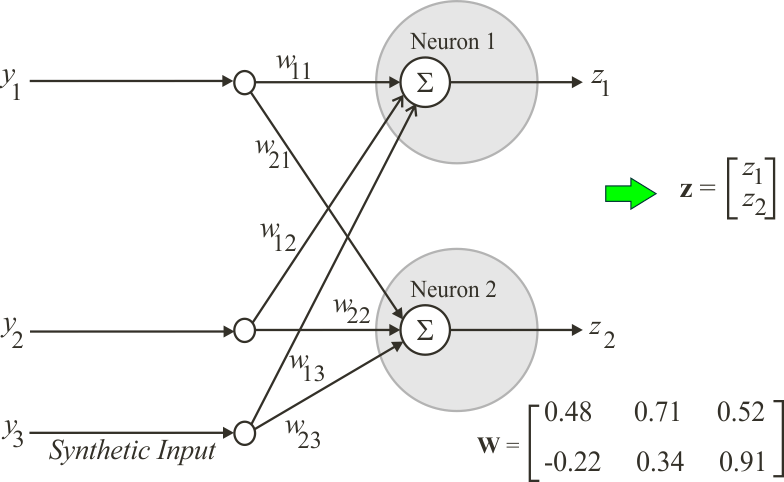Problem 2 Repeat last problem using Neural Lab Code called your project Unsuper (use the Main file only option). First, use notepad to create the files as shown. Second, edit the Main.lab file with the code shown below and then execute it.